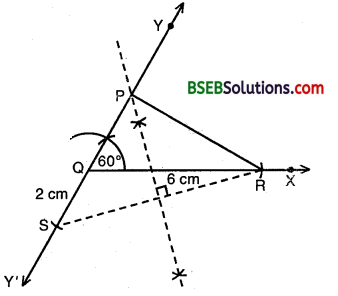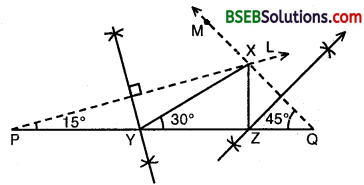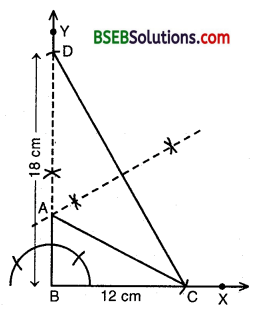# Bihar Board Class 9th Maths Solutions Chapter 11 Constructions Ex 11.2

Bihar Board Class 9th Maths Solutions Chapter 11 Constructions Ex 11.2 Textbook Questions and Answers.

## BSEB Bihar Board Class 9th Maths Solutions Chapter 11 Constructions Ex 11.2Question 1.
Construct a triangle ABC in which BC = 7 cm, ∠B = 75° and AB + AC = 13 cm.
Solution:
Steps of Construction

1. Draw a ray BX and cut off a line segment BC = 7 cm.
2. Construct ∠XBY = 75°.3. From BY, cut off BD = 13 cm.
4. Join CD.
5. Draw the perpendicular bisect of CD, intersecting BA at A.
6. Join AC.

The triangle ABC thus obtained is the required triangle.Question 2.
Construct a triangle ABC in which BC = 8 cm, ∠B = 45° and AB – AC = 3.5 cm.
Solution:
Steps of Construction :

1. Draw a ray BX and cut off a line segment BC = 8 cm from it.
2. Construct ∠YBC = 45°3. Cut off a line segment BD = 3.5 cm from BY.
4. Join CD.
5. Draw per-pendicular bisector of CD intersecting BY at a point A.
6. Join AC.

Then, ABC is the required triangle.Question 3.
Construct a triangle PQR in which QR = 6 cm, ∠Q = 60° and PR – PQ = 2 cm.
Solution:
Steps of Construction :

1. Draw a ray QX and cut off a line segment QR = 6 cm from it.
2. Construct a ray QY making an angle of 60° with QR and produce YQ to form a line YQY’.3. Cut off a line segment QS = 2 cm from QY’.
4. Join RS.
5. Draw perpendicular bisector of RS intersecting QY at a point P.
6. Join PR.

Then, PQR is the required triangle.Question 4.
Construct a triangle XYZ in which ∠Y = 30°, ∠Z = 90° and XY + YZ + ∠X = 11 cm.
Solution:
Steps of Construction :

1. Draw a line segment PQ = 11 cm.
2. At P, draw a ray PL such ∠LPQ = $$\frac { 1 }{ 2 }$$ x 30° = 15°3. At Q, draw ray QM such that ∠MQP = $$\frac { 1 }{ 2 }$$ x 90° = 45° intersecting PL at X.
4. Draw perpendicular bisectors of XP and XQ intersecting PQ in Y and Z respectively.

Then, ∆ XYZ is the required triangle.

Note : For clarity in figure, method of drawing angles of 15° and 45° have not been shown. Students should draw these angles with the help of ruler and compass only by the method as shown earlier.Question 5.
Construct a right triangle whose base is 12 cm and sum of its hypotenuse and other side is 18 cm.
Solution:
Steps of Construction :

1. Draw a ray BX and cut off a line segment BC = 12 cm.
2. Construct ∠XBY = 90°.3. From By cut off a line segment BD = 18 cm.
4. Join CD.
5. Draw the per¬pendicular bisector of CD intersecting BD at A.
6. Join AC.

Then ABC is the required triangle.